• 输入算符优先文法，输出FIRSTVT、LASTVT、算符优先关系表 对输入串，输出分析过程
• 算符优先法中算符优先矩阵的构造需要求出firstTerm以及lastTerm，由于这个是在预测分析法上进行修改的，结构体的定义并未进行修改，其中包含该非终结符a以及其对应的first（即为firstTerm）和follow（即为lastTerm）...

算符优先法中算符优先矩阵的构造需要求出firstTerm以及lastTerm，由于这个是在预测分析法上进行修改的，结构体的定义并未进行修改，其中包含该非终结符a以及其对应的first（即为firstTerm）和follow（即为lastTerm），输入格式如下（以#结束输入）
Z::=E
E::=T|E+T
T::=F|T*F
F::=(E)|i
#

#include<bits/stdc++.h>
using namespace std;
//判断终结符和非终结符 返回1是非终结符 返回0是终结符
int Norterminal(char c)
{
if(c>='A'&&c<='Z')
return 1;
else if(c!=':'&&c!='='&&c!='<'&&c!='>'&&c!=' '&&c!='|')
return 0;

}
struct GRAM
{
//$代表空 string a; string first; string follow; }; struct LIST { int num; string s; }; int main() { GRAM gram; string grammer; cout<<"请输入文法（以#结束）"<<endl; std::vector<string> strN; cin>>grammer;//输入规则 strN.push_back(grammer); char str; const char *ch = "|"; char *result; vector<string> strN1; //存处理过“|”规则的 for(int i=0;i<grammer.length();i++) { str[i]=grammer[i]; } for(int h=grammer.length();h<80;h++) { str[h]=NULL; } result = strtok(str,ch); while(result!=NULL) { strN1.push_back(result); result = strtok(NULL,ch); } for(int i=1;grammer!="#";i++) { cin>>grammer; strN.push_back(grammer); /*处理转换的规则形式输入部分*/ for(int h=0;h<grammer.length();h++) { str[i][h]=grammer[h]; } for(int h=grammer.length();h<80;h++) { str[i][h]=NULL; } result = strtok(str[i],ch); while(result!=NULL) { strN1.push_back(result); result = strtok(NULL,ch); } } /* 获取firstTerm集合 */ //直接获取firstTerm for(unsigned i=0;i<strN.size();i++) { gram[i].a=strN[i]; gram[i].first=""; if(!Norterminal(strN[i])) { gram[i].first=strN[i]; } else { if(!Norterminal(strN[i])) { gram[i].first=strN[i]; } } for(unsigned k=5;k<strN[i].length();k++) { if(strN[i][k]=='|') { if(!Norterminal(strN[i][k+1])) { gram[i].first+=strN[i][k+1]; } else { if(!Norterminal(strN[i][k+2])) { gram[i].first+=strN[i][k+2]; } } } } } int num=strN1.size(); //当第一个字符是非终结符时 for(int i=num-1;i>=0;i--) { for(unsigned l=0;l<strN.size()-1;l++) { if(gram[l].a==strN1[i]) { for(unsigned h=0;h<strN.size()-1;h++) { if(strN1[i]==gram[h].a&&strN1[i]!=strN1[i]) { gram[l].first+=gram[h].first; string ustr(gram[l].first); sort(ustr.begin(), ustr.end()); ustr.erase(unique(ustr.begin(), ustr.end()), ustr.end() ); gram[l].first=ustr; break; } } } } } cout<<"firstTerm集合为："<<endl; for(unsigned i=0;i<strN.size()-1;i++) { for(int k=0;k<gram[i].first.length();k++) { if(gram[i].first[k]==NULL) { gram[i].first.erase(gram[i].first.begin()+k); } } cout<<gram[i].a<<" "<<gram[i].first<<endl; } string term=""; cout<<"终结符为："<<endl; for(unsigned i=0;i<strN.size()-1;i++) { for(int j=0;j<strN[i].length();j++) { if(!Norterminal(strN[i][j])&&strN[i][j]!='$')
term+=strN[i][j];
}
string ustr(term);
sort(ustr.begin(), ustr.end());
ustr.erase(unique(ustr.begin(), ustr.end()), ustr.end() );
term=ustr;
}
cout<<term<<endl;
/*转换规则形式*/
cout<<"转换后的规则形式为："<<endl;
int listnumber =0;
for(unsigned i=0;i<strN1.size()-1&&i-1>=0;i++)
{

if(strN1[i].find("::")==-1)
{

}
cout<<strN1[i]<<endl;
}
/*获取lastTerm*/
//直接获取lastTerm
for(int i=0;i<num-1;i++)
{
for(unsigned l=0;l<strN.size()-1;l++)
{
if(gram[l].a==strN1[i])
{
for(unsigned h=0;h<strN.size()-1;h++)
{
int k=strN1[i].length();
if(!Norterminal(strN1[i][k-1]))
{

gram[l].follow+=strN1[i][k-1];
string ustr(gram[l].follow);
sort(ustr.begin(), ustr.end());
ustr.erase(unique(ustr.begin(), ustr.end()), ustr.end() );
gram[l].follow=ustr;
break;
}
else
{
if(!Norterminal(strN1[i][k-2]))
{
gram[l].follow+=strN1[i][k-2];
string ustr(gram[l].follow);
sort(ustr.begin(), ustr.end());
ustr.erase(unique(ustr.begin(), ustr.end()), ustr.end() );
gram[l].follow=ustr;
break;
}
}
}
}
}
}
for(int i=num-1;i>=0;i--)
{
for(unsigned l=0;l<strN.size()-1;l++)
{
if(gram[l].a==strN1[i])
{
for(unsigned h=0;h<strN.size()-1;h++)
{
int k=strN1[i].length();
if(Norterminal(strN1[i][k-1]))
{

for(unsigned h1=0;h1<strN.size()-1;h1++)
if(gram[h1].a==strN1[i][k-1])
{
gram[l].follow+=gram[h1].follow;
string ustr(gram[l].follow);
sort(ustr.begin(), ustr.end());
ustr.erase(unique(ustr.begin(), ustr.end()), ustr.end() );
gram[l].follow=ustr;
break;
}

}
}
}
}

}
cout<<"lastTerm集合为："<<endl;
for(unsigned i=0;i<strN.size()-1;i++)
{
cout<<gram[i].a<<" "<<gram[i].follow<<endl;
}
int list[term.length()][term.length()];
for(int i=0;i<term.length();i++)
{
for(int j=0;j<term.length();j++)
{
list[i][j]=0;
}
}
cout<<"相等的关系为："<<endl;
for(int i=num-1;i>=0;i--)
{
for(int k=0;k<strN1[i].length()&&k+1<strN1[i].length();k++)
{
if(Norterminal(strN1[i][k]))
{
if(!Norterminal(strN1[i][k-1])&&!Norterminal(strN1[i][k+1]))
{
cout<<strN1[i][k-1]<<strN1[i][k+1]<<endl;;
for(int h=0;h<term.length();h++)
{
if(strN1[i][k-1]==term[h])
for(int g=0;g<term.length();g++)
{
if(strN1[i][k+1]==term[g])
{
list[h][g]=1;
}
}
}
}
}
else
{
if(!Norterminal(strN1[i][k+1]))
{
cout<<strN1[i][k]<<strN1[i][k+1]<<endl;
for(int h=0;h<term.length();h++)
{
if(strN1[i][k]==term[h])
for(int g=0;g<term.length();g++)
{
if(strN1[i][k+1]==term[g])
{
list[h][g]=1;
}
}
}
}
}
}
}
//cout<<"小于关系为："<<endl;
for(int i=0;i<num-1;i++)
{
for(int k=0;k<strN1[i].length();k++)
{
if(!Norterminal(strN1[i][k]))
{
if(Norterminal(strN1[i][k+1]))
{
for(int h=0;h<term.length();h++)
{
if(strN1[i][k]==term[h])
{
for(int j=0;j<num-1;j++)
{
if(strN1[i][k+1]==gram[j].a)
{
for(int g=0;g<term.length();g++)
{
for(int f=0;f<gram[j].first.length();f++)
if(gram[j].first[f]==term[g])
{
list[h][g]=2;
}
}
}
}
}
}

}
}
}

}
//大于关系
for(int i=0;i<num-1;i++)
{
for(int k=0;k<strN1[i].length();k++)
{
if(Norterminal(strN1[i][k]))
{
if(!Norterminal(strN1[i][k+1]))
{
for(int h=0;h<term.length();h++)
{
if(strN1[i][k+1]==term[h])
for(int j=0;j<num-1;j++)
{
if(strN1[i][k]==gram[j].a)
{
for(int g=0;g<term.length();g++)
{
for(int f=0;f<gram[j].first.length();f++)
if(gram[j].follow[f]==term[g])
{
list[g][h]=3;
}
}
}
}
}

}
}
}
}
for(int i=0;i<term.length();i++)
{
for(int j=0;j<term.length();j++)
{
cout<<list[i][j]<<" ";
}
cout<<endl;
}

cout<<"算符优先矩阵为："<<endl;
cout<<"    |";
for(int i=0;i<term.length();i++)
{
cout<<setw(10)<<term[i];
cout<<"|";
}
cout<<endl;
cout<<"____________________________________________________________________________"<<endl;

for(int i=0;i<term.length();i++)
{
printf("%2c",term[i]);
cout<<"  "<<"|";
for(int j=0;j<term.length();j++)
{
if(list[i][j]==0)
cout<<setw(10)<<" "<<"|";
if(list[i][j]==1)
cout<<setw(10)<<"="<<"|";
if(list[i][j]==2)
cout<<setw(10)<<"<"<<"|";
if(list[i][j]==3)
cout<<setw(10)<<">"<<"|";

}
cout<<endl;
cout<<"____________________________________________________________________________"<<endl;

}

}

运行结果如下：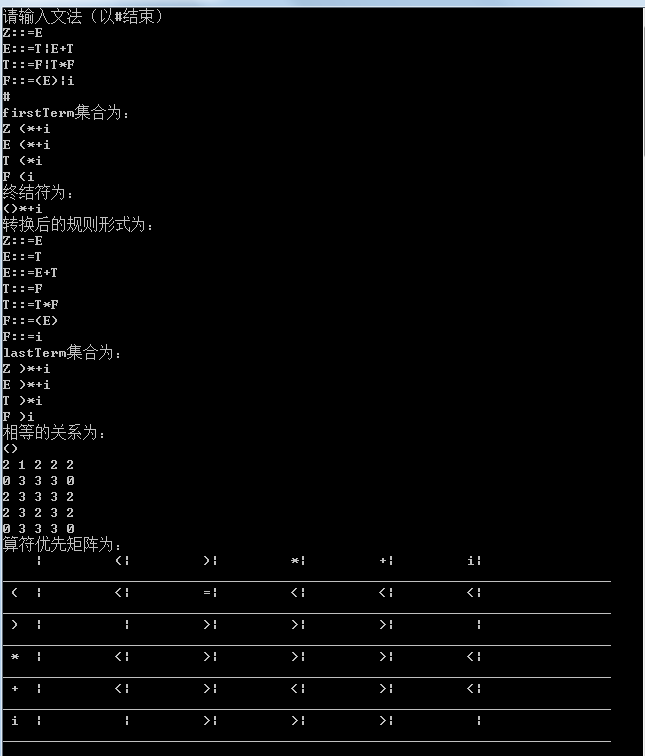展开全文• 编译原理：算符优先分析法

千次阅读 2020-05-28 08:22:45
编译原理：算符优先分析文法

编译原理：算符优先分析法

语法分析有自上而下（推导）和自下而上（归约）分析法两种，而算符优先分析法是自上而下分析法中的一种，应用也比较普遍，但是值得注意的是，其不是规范归约（最左归约）。

一般情况，算符优先分析法的流程如下：
求解FIRSTVT集和LASTVT集→构造优先关系矩阵→进行句型的“归约”操作。

下文所用文法如下：
E→E+T |T
T→T*F | F
F→(E) | i

FIRSTVT集和LASTVT集

这里我们需要与FIRST集和FOLLOW集合做一下区分：
（1）FIRST集和FOLLOW集：
①用于LL(1)文法的预测分析表构造
②FIRST集和FOLLOW集中均为终结符号集合，但是FOLLOW集中没有ε
（2）FIRSTVT集和LASTVT集：
①用于算符优先文法中的优先关系矩阵的构造
②FIRSTVT集和LASTVT集均为终结符号集合

FIRSTVT集

*定义+求法：通过定义我们可以得出以下几点：
① U→b…，b进入FIRSTVT(U)
②U→V…，FIRSTVT(V)进入FIRSTVT(U)
③U→Vb…，b进入FIRSTVT(U)

（注意：U和V：非终结符号；b：终结符号）

• 例解
FIRSTVT(E)={+,* , ( , i }
FIRSTVT(T)={ * , ( , i }
FIRSTVT(F)={ ( , i }

LASTVT集

• 定义+求法：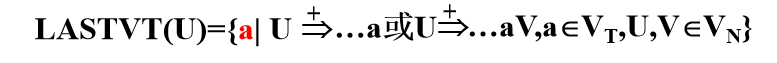通过定义我们可以得出以下几点：
①U->…a，a进入LASTVT（U）
②U->…V，LASTVT（V）进入LASTVT（U）
③U->…aV，a进入LASTVT（U）

（注意：U和V：非终结符号；a：终结符号）

• 例解
LASTVT(E)={+ , * , ) , i }
LASTVT(T)={ * , ) , i }
LASTVT(F)={ ) , i }

优先关系矩阵

如果要构造优先关系矩阵，我们需要明确两个相继出现的终结符号a和b之间的优先关系(a与b之间可有一个非终结符)，一旦确定了这种优先关系，就可以用它来确定“可归约串”进行归约。

①a·>b（a的优先级高于b的优先级）：当且仅当G中含有产生式：U→…Ub…，且任意的a∈LASTVT（U），a·>b成立。
②a<·b（a的优先级低于b的优先级）：当且仅当G中含有产生式：U→…aU…，且任意的b∈FIRSTVT（U），a<·b成立。
③a=·b（a的优先级等于b的优先级）：当且仅当G中含有产生式：U→…ab…或U→aVb…

NOTE：
①优先级高的先参加归约！
②优先关系不是对偶的，也就是说：a<·b≠b·>a

• 例解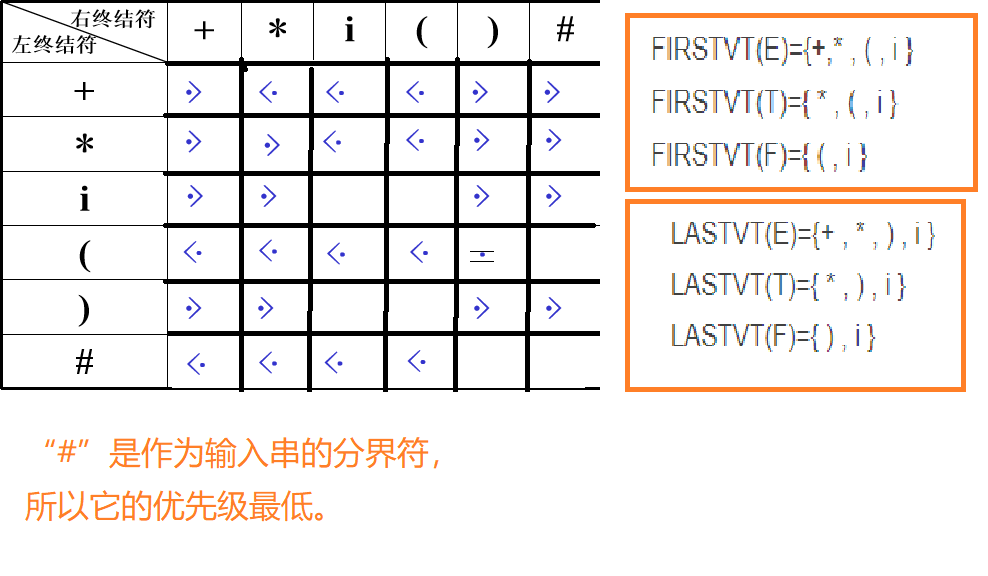归约

在算符优先分析法中采用的是归约最左素短语的方式进行归约。

预备知识

• 概念问题

（1）短语：一棵子树的所有叶子自左至右排列起来形成一个相对于子树根的短语。
（2）直接短语：仅有父子两代的一棵子树，其所有叶子自左至右排列起来所形成的符号串。
（3）句柄：一个句型的分析树中最左那棵只有父子两代的子树的所有叶子的自左至右排列。
（3）素短语：至少包含有一个终结符号，并且除它之外，不再包含其它素短语。
（4）最左素短语：任意句型最左边的素短语为该句型的最左素短语。
举例：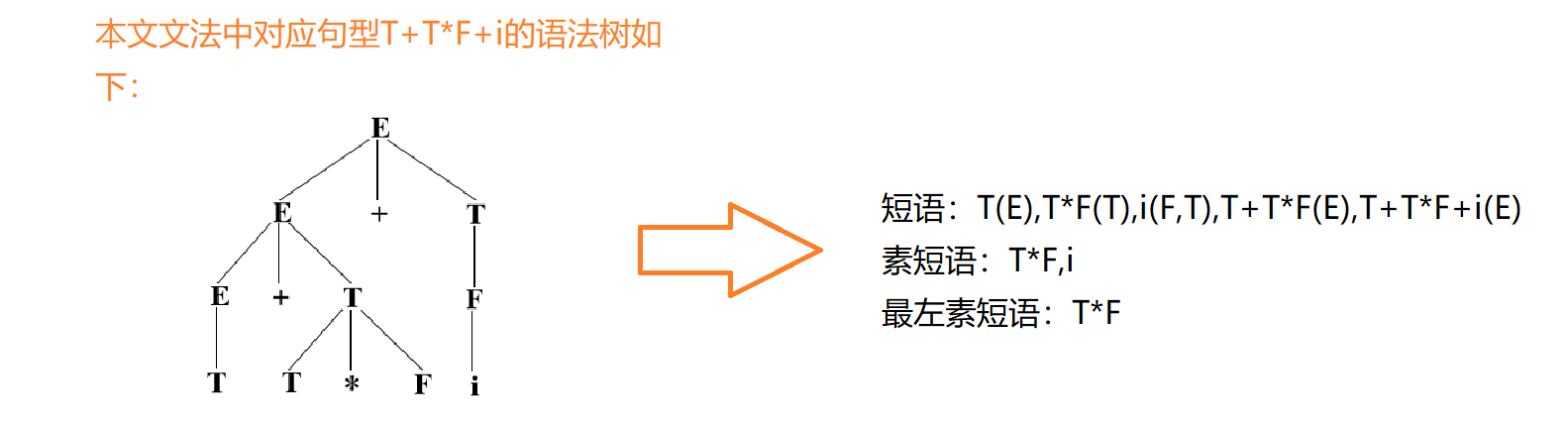• 最左素短语的特征
（1）算符文法句型的一般形式：#N1a1N2a2……NnanNn+1# 其中，Ni∈VN, ai∈VT；
（2）定理：一个算符优先文法的句型的最左素短语是满足下列条件的最左子串：NjajNj+1aj+1……NiaiNi+1

其优先关系为：aj=.aj+1 ，aj+1=. aj+2, …, ai-1 =. ai；且：aj-1<.aj， ai >. ai+1

将子串左右邻接符号一起考虑：aj-1NjajNj+1aj+1……NiaiNi+1ai+1
它们的优先关系表示为：aj-1<.aj=. aj+1=. ……=.ai>.ai+1

则<.和>.之间用等号连接的部分（aj=. aj+1=. ……=.ai）即为最左素短语，可以进行归约。

注意：
Nj和Ni+1属于最左素短语，而aj-1和ai+1 不属于最左素短语。

• 例解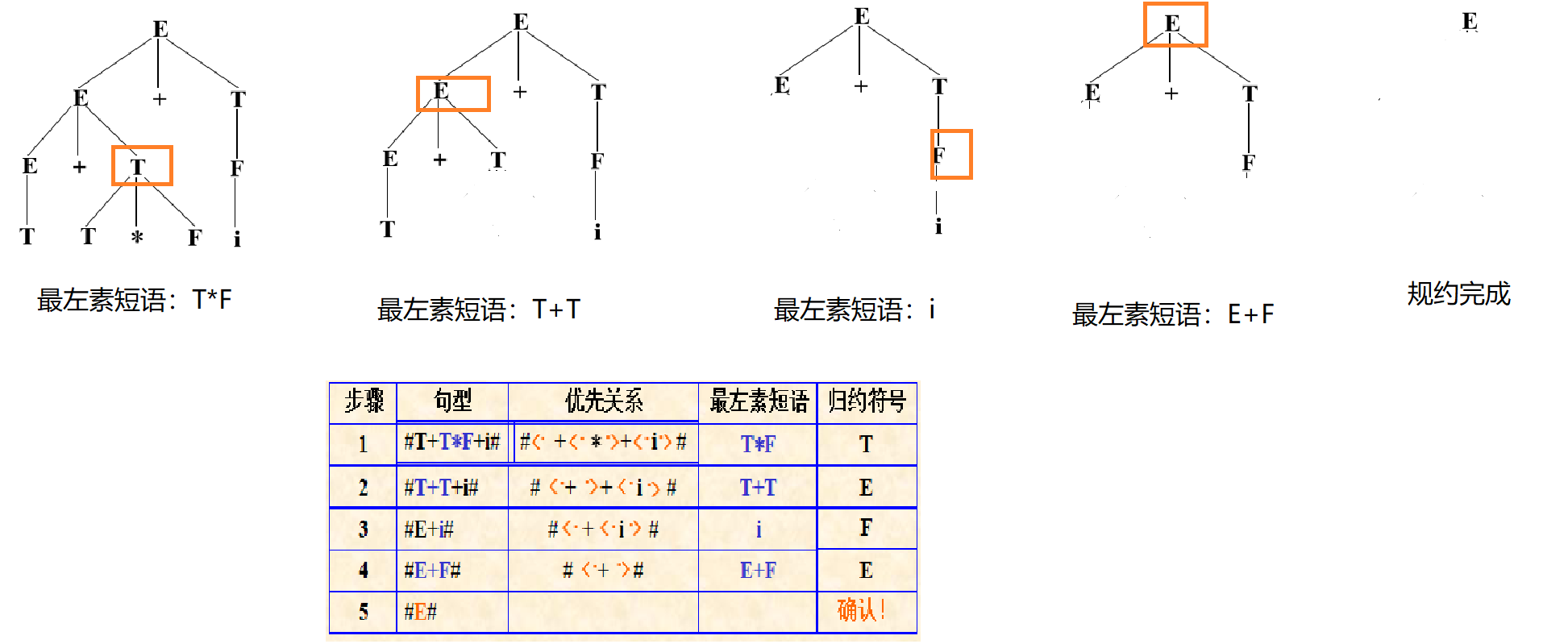至此，算符优先分析已经完成。
展开全文• 编译原理之算符优先分析

千次阅读 2019-06-20 11:57:29
2. 构造优先关系矩阵的算法？举例加以讲解；四. 算符优先分析法最左规约串的确定1. 最左素短语的定义是什么？2. 最左素短语的特征？如何根据其特征确定当前句型的最左可归约串？3. 什么是“单非产生式”，算符优...

一. 什么是算符文法？应满足什么条件

• 算符文法
设有一个文法G，若G中有形如U->Vw的产生式，即它的任意产生式的右部都不含两个相继的非终结符，则称G为算数文法，或称为OG文法。

• 算符优先文法
（1）是自上而下分析法中的一种，虽然他不是规范规约，但具有分析速度快的特点，是和表达式分析。
（2）算符优先分析法就是仿照算数四则运算的运算过程。定义任意两个相继出现的终结符号a和b之间的优先关系，一点确定了这种优先关系，就可以用他确定“句柄”进行规约。

二. 算符文法中，相邻算符之间的优先关系有几种？如何定义？

设G是不含ε－产生式的文法，对任何终结符a,b∈VT，A,B,C∈VN

1. a优先级高于b： a > b，a先于b被规约
2. a优选级等于b： a = b，a与b同时被规约
3. a优先级低于b： a < b，a后于b被规约

三. 如何构造优先关系矩阵？

1. FIRSTVT集、LASTVT集的定义及构造方法；

定义FirstVT§={a|P(+=>)a···或P(+=>)Qa···，a属于终结字符集，而Q属于非终结字符集}，其中···表示所有字符集
LastVT（P）={a|P(+=>)···a或P(+=>)···aQ，a属于终结字符集，而Q属于非终结字符集}

• 由以下两条规则来构造FirstVT集：
1. 若有产生式P=>a···、或P=>Qa···，则a属于FirstVT（P）；
2. 若有a属于FirstVT（Q），且有产生式P=>Q···，则a属于FirstVT§;# 四. 算符优先分析法最左可规约串的确定
• 类似的有构造LastVT集的规则：
1. 若有产生式P=>···a或P=>···aQ，则a属于LastVT集。
2. 若a属于LastVT（Q），且有产生式P=>···Q，则a属于LastVT（P）集。

2. 构造优先关系矩阵的算法？举例加以讲解；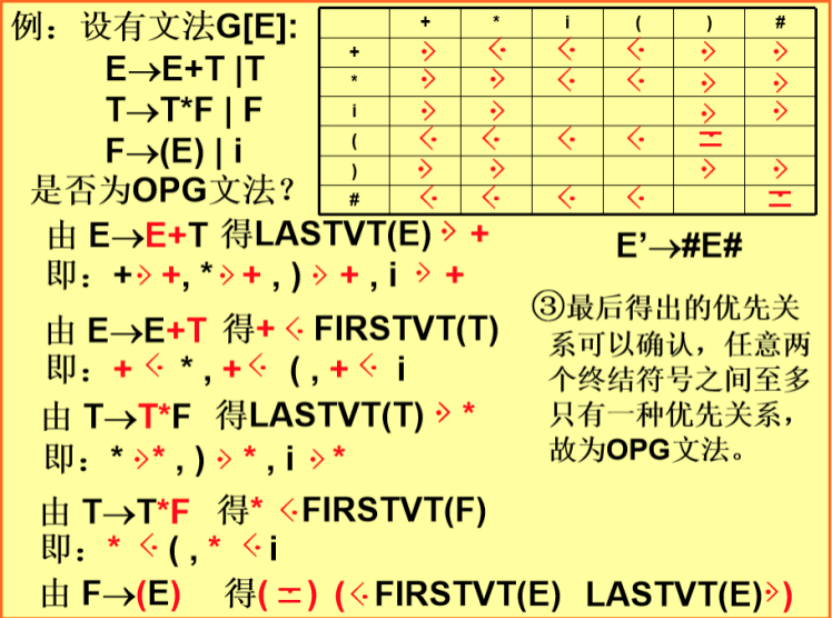四. 算符优先分析法最左规约串的确定

1. 最左素短语的定义是什么？

• 素短语：某文法句型的短语它至少包含有一个终结符号，并且除它之外，不再包含任何更小的素短语。
• 最左素短语：任意句型最左边的素短语。

2. 最左素短语的特征？如何根据其特征确定当前句型的最左可归约串？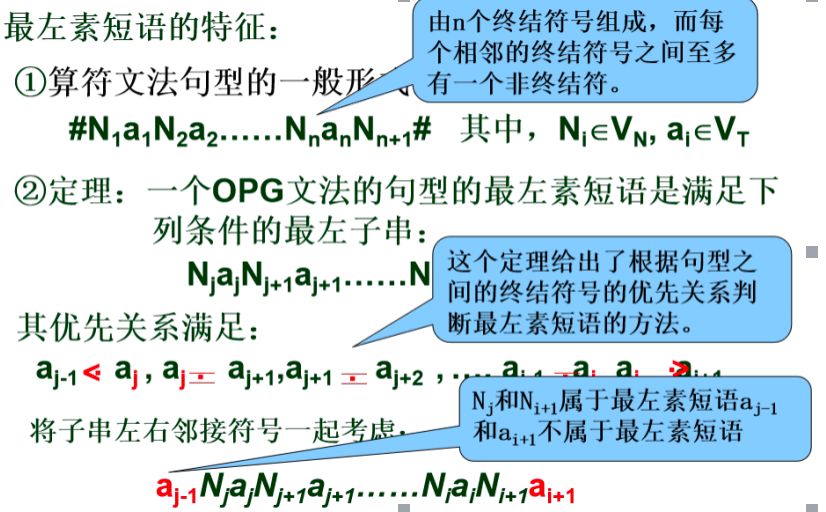3. 什么是“单非产生式”，算符优先分析法进行规约为什么速度快？

• 右部仅有一个非终结符的产生式
• 比用文法的优先关系矩阵节省内存，若有n个终结符号，优先关系矩阵占内存为(n+1)2，优先函数为2(n+1);
• 编程时便于比较运算，即用一般的关系运算即可;

五. 算符优先分析法总控程序的工作流程及伪代码

1. 工作流程

1. 定义两个相继出现的终结符a 和b 的优先关系
2. 确定要规约的句型的最左素短语
3. 将最左素短语规约

2. 伪代码

void Isleft( )
{  Stack s;
k=1;
s[k]=’#’;
do{   把下一个输入符号读进a中；
if (S[k]∈VT)   j=k;
else                j=k-1;
while(S[j]>a)
{  do{    Q=S[j];
if(S[j-1] ∈VT)   j=j-1;
else                   j=j-2;
}while(S[j]>Q);
把S[j+1]…S[k]归约为某个N；
k=j+1;
S[k]=N;
}
if(S[j]<a || S[j]=a)
{   k=k+1;
S[k]=a;
}
}while(a!=’#’);
}

展开全文编译原理 算符优先分析
• 算符优先法之优先表构造

千次阅读 2019-07-02 11:27:00
算符优先分析不是一种规范...对于任何两个可能相继出现的终结符 a 和 b ，它们之间的优先关系无非 >、=、<三种关系。 需要注意的是a<b，并不意味着 b > a，a=b并不意味着 b = a 。这是因为与它们在规...

算符优先分析不是一种规范规约法，但是该方法特别有利于表达式分析，宜于手工实现。

算符优先分析法和计算的过程相同，由此判断一个符号的左右符号优先级，从而确定是否可以规约。

对于任何两个可能相继出现的终结符 a 和 b ，它们之间的优先关系无非 >、=、<三种关系。

需要注意的是a<b，并不意味着 b > a，a=b并不意味着 b = a 。这是因为与它们在规约的前后相对位置也有非常大的关系。这在之后构造的算符优先表中可以看出。

要构造算符优先表。先要搞清楚两个集合的概念：

1、FIRSTVT(P) ={ a | P =>a……或 P=>Qa……，a为终结符，Q为非终结符 }

2、LASTVT(P)={ a | P=>……a 或 P=>……aQ ,a为终结符，Q为非终结符 }

构造FIRSTVT集的方法

（1）、若有产生式P =>a……或 P=>Qa……，则 a 属于FIRSTVT(P)

（2）、若 a 属于FIRSTVT(Q) , 且有产生式 P=>Q…… ,则 a 属于 FIRSTVT(P)

同理构造相应的LASTVT集。

有了这两个集合，我们根据下面三条准则来判断两个终结符直接的算符优先关系。

（1）、a=b ，当且仅当文法G中含有形如 P=>……ab……或 P=>……aQb……的产生式；

（2）、a<b，当且仅当G中含有形如 P=>……aR……，而 R =>b……或 R =>Qb……;

（3）、a>b，当且仅当G中含有形如 P=>……Rb……的产生式，而R=>……a 或 R=>……aQ。

由此可以构造出优先表。

转载于:https://www.cnblogs.com/ISGuXing/p/11119515.html

展开全文• 算符优先分析法中，文法终结符之间的优先关系是用优先矩阵表示的，这样需要占用大量的内存空间，当文法有n个终结符时，就需要（n+1）^2个内存单元，因此，在实际实现中使用优先函数来代替优先矩阵表示优先关系。...
• 最易于实现的一种移动归约分析方法，叫做算符优先分析法，而更一般的移动归约分析方法叫做LR分析法，LR分析法可以用作许多自动的语法分析器的生成器。 短语：文法G[S]，αβδ是文法G的一个句型，S=>*αAδ且A=>+β...
• 算符优先分析法 1.基本概念 算符文法(OG)：文法G中没有形如A=&gt;···BC···的产生式，其中B、C为非终结符，则G为算符文法（operator grammar）。 也就是说产生式的右部不能出现两个非终结符相邻，就...
• （1）根据给定文法，先求出FirstVt和LastVt集合，构造算符优先关系表（要求算符优先关系表 输出到显示器或者输出到文件）； （2）根据算法和优先关系表分析给定表达式是否是该文法识别的正确的算术表达式（要求输出...编译原理 语法分析 算符优先分析 C++
• 用C++编译的算符优先数.利用算符优先分析方法和思想对某些语句进行语法分析与语义分析，生成相应的中间代码。学会正确运用语法规则，并能应用所学的方法解决存在的问题。给出语法分析方法及中间代码形式的描述、文法...
• Firstvt 找Firstvt的三条规则：如果要找A的Firstvt，A的候选式中出现： A->a…，即以终结符开头，该终结符入Firstvt A->B…，即以非终结符开头，该非终结符...前面是终结符，则终结符入Firstvt 算符优先关系表 实例 2.
•编译原理 算符优先 优先函数
• java实现算符优先文法ll1文法

千次阅读 2019-06-04 21:22:27
实验要求： 1.[实验项目] 实现LL(1)分析中控制程序（表驱动程序）；完成以下描述赋值语句的LL(1)文法的LL(1...（1）构造该算符优先文法的优先关系矩阵或优先函数； （2）输入串应是词法分析的输出二元式序列，即某算...编译原理 算符优先文法 java
• 自底向上分析之算符优先分析法 说明：以老师PPT为标准，借鉴部分教材内容，AlvinZH学习笔记。 基本过程 1. 一般方法：采用自左向右地扫描和分析输入串，从输入符号串开始，通过反复查找当前句型的句柄（最左简单短语...
• 算符优先语法分析程序

热门讨论 2012-07-07 21:27:39
（1）构造该算符优先文法的优先关系矩阵或优先函数； （2）输入串应是词法分析的输出二元式序列，即某算术表达式“实验项目一”的输出结果。输出为输入串是否为该文法定义的算术表达式的判断结果。 （3）算符优先...
• System.out.println("算符优先关系矩阵"); System.out.println("-------------------------------------------------------------------"); System.out.print("\t"); for(int i=0;i;i++){ System.out.print(T...
• 算符优先分析

2021-03-09 19:07:14
1. 已知算符优先关系矩阵如下表：+*i()#+><<<>>*>><<>>i>>>>(<<<<=)>>>>#<<<<=写出符号串(i+i)*i#的算符优先分析过程。2.接...
• 所谓算符优先分析法就是依照算术表达式的四则运算过程而设计的一种语法分析方法。 这种分析方法首先要规定运算符之间(确切地说是终结符之间)的优先关系和结合性质,然后借助这种关系,比较相邻运算符的优先级来确定...
• 算符优先文法

千次阅读 2020-11-28 16:40:58
算符优先文法概述自底向上分析优先关系算符文法算符优先文法构造算符优先表的算法确定算符优先关系构造集合FIRSTVT(P)FIRSTVT(P)FIRSTVT(P)的算法构造集合LASTVT(P)LASTVT(P)LASTVT(P)的算法构造算符优先表实例算符...编译器
• 算符优先分析法-思路方法在这里

千次阅读 多人点赞 2020-05-16 20:48:28
3.构造并输出算符优先分析表，判断是否为算符优先文法，若不是提示无法进行分析； 4.任意输入一个输入串，可得到成功的分析或错误的提示，输出其分析过程或打印语法 分析树。 实验运行结果 算符优先文法的特点： ...编程语言 算法
• 算符优先分析的步骤 构造优先关系矩阵（通过FIRSTVT和LASTVT集构造） 构造一个输入串（要在两边加上#）和一个符号栈 当栈内首个终结符的优先级或＝栈外终结符的优先级时，移进；当栈内首个终结符的优先级＞栈外终结...算法 java 编译器 数据结构
• 若已知运算符之间的优先关系，可按如下步骤构造优先函数： 1、对每个运算符a（包括#在内）令f(a)=g(a)=1 2、如果a⋗b且f(a)<=g(b)令f(a)=g(b)+1 3、如果a⋖b且f(a)>=g(b)令g(b)= f(a)+1 4、如果a≐b而f(a)...
• 2）采用由定义构造算符优先关系集合并构造矩阵 由以上公式，先得到对应的FIRSTVT与LASTVT集合，再依次遍历文法找到所需的…aV…或者…Vb…产生式，与FIRSTVT（V）或者LASTVT（V）卡氏积，得到>,<,=的算符优先...java
• 【编译原理】算符优先算法

万次阅读 多人点赞 2018-05-10 20:48:55
G[E]:E→E+T∣T T→T*F∣FF→(E)∣i构造该算符优先文法的优先关系矩阵或优先函数；输入串应是词法分析的输出二元式序列，即某算术表达式“实验项目一”的输出结果。输出为输入串是否为该文法定义的算术表达式的判断...
• 内含代码片段。原理包括算符优先的三种优先关系定义与判断方法，FIRSTVT集和LASTVT集的构造步骤；判断算符关系，构造算符优先关系矩阵的说明；根据矩阵分析句子合法性的步骤说明；实验结果包含输入与输出
• 算符优先分析法-java实现

千次阅读 多人点赞 2019-12-03 17:50:33
算符优先分析器-java实现 一、判断是否是算符文法 算符文法定义:一个文法，如果它的任一产生式的右部都不含两个相继(并列)的非终结符，即不含如下形式的产生式右部:…QR…,则称该文法为算符文法 二、求Firstvt和...算符优先分析器 java
• https://github.com/Ggmatch/The-principle-to-compile体验算法优先分析法能达到的效果算符优先分析法只考虑算符（广义为终结符）之间的优先关系，例如若有文法G为： （1）E->E+E （2）E->E*E （3）E->编译原理
• 首先看一下什么是算符文法 算符文法 设有文法G，若G中没有形如U —> …VW…的规则，其中VW为非终结符，则称G为...定义任何两个终结符号之间的优先关系 设G是一个算符文法，a和b是任意两个非终结符，P、Q、R是非......